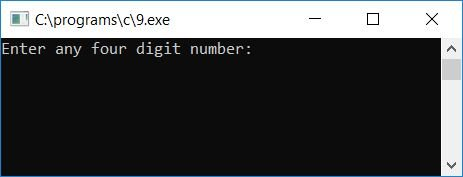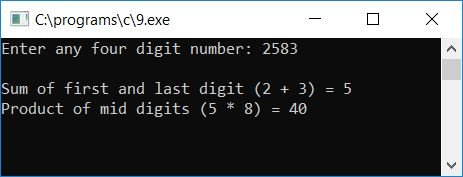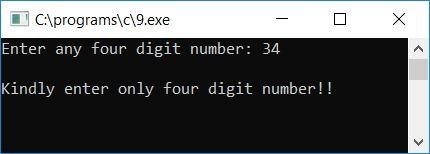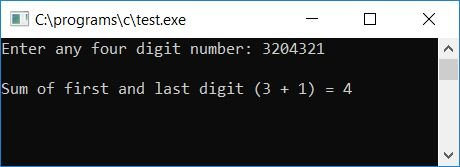# C program to find the sum of the first and last digits, the product of the middle digits

In this article, we will learn how to create a program in C that will ask the user to enter any 4-digit number to find and print the sum of its first and last digits and then the product of its middle digit. For example, if the user enters 8427 as input, then the program will calculate the sum of the first and last digits, which will be 8+7 or 15, and then the product of the mid-digit, which will be 4*2 or 8.

After this program, we have also created another that will find and print the sum of the first and last digits of any two- or more-digit number given by the user. But let's first take a look at the program given here to learn how to calculate and print the sum of the first and last digits and the product of the mid-digits of a 4-digit number.

```#include<stdio.h>
#include<conio.h>
int main()
{
int num, first, last, second, third, prod, sum;
int rem, count=1, temp, tempcount=0;
printf("Enter any four digit number: ");
scanf("%d", &num);
temp = num;
while(temp>0)
{
tempcount++;
temp = temp/10;
}
if(tempcount==4)
{
while(num>0)
{
rem = num%10;
if(count==1)
first = rem;
else if(count==2)
second = rem;
else if(count==3)
third = rem;
else if(count==4)
last = rem;
num = num/10;
count++;
}
sum = first+last;
prod = second*third;
printf("\nSum of first and last digit (%d + %d) = %d", last, first, sum);
printf("\nProduct of mid digits (%d * %d) = %d", third, second, prod);
}
else
printf("\nKindly enter only four digit number!!");
getch();
return 0;
}```

As the program was written in the Code::Blocks IDE, therefore, after a successful build and run, you will get the output as shown in the snapshot given here:Now supply any 4-digit number, say 2583, as input and press the ENTER key to see the output as given here:Let's check the above program with another sample run to see what will happen if the user supplies any number that is not a 4-digit number, say 34. Here is the sample run:#### Program Explained

• Receive any 4-digit number as input.
• Now initialize the number to any variable, say temp.
• Create a while loop that runs until the value of temp is greater than 0.
• Inside the while loop, increment any variable, say tempcount, and divide temp with 10.
• Never forget to initialize 0 to tempcount at the start of the program.
• Here, using the while loop, we have counted the number digit-by-digit to check whether the given number is a 4-digit number or not.
• That is, if the user enters 2583 as input, then num holds this entered value.
• The value of num gets initialized to temp. Now, temp is 2583.
• As the value 2583 is greater than 0, therefore the program flow goes inside the while loop and increments the value of tempcount, and the initial value of  tempcount is 0, therefore at the first run of the while loop, tempcount holds its value of 1, and temp/10 or 2583/10 or 258 is initialized to temp.
• At the second run of the while loop, as 258 is greater than 0, program flow returns to the while loop, and tempcount is incremented to 2, and temp/10, 258/10, or 25 is initialized to temp.
• At the third run, follow the same process and continue doing so until the temp holds its value of 0.
• After exiting the while loop, the variable tempcount holds the value that represents the total number of digits present in that given number as entered by the user at run-time. That is, if the user enters 234, then tempcount holds its value as 3 (as it is a 3-digit number) after running and exiting from the while loop.
• Now check whether the tempcount is equal to 4 or not.
• If it is, then the given number is a 4-digit number.
• And if it is a 4-digit number, then follow the next step to calculate and print the sum of its first and last digits and the product of its mid-digits (second and third digits).
• Otherwise, if it is not a 4-digit number, then print a message like "Please enter a 4-digit number" and exit from the program.
• To calculate the sum of its first and last digits and the product of its middle digit, follow the steps given below.
• Use a while loop to run until the value of num equals zero.
• Inside the loop, find its digit using the modulus or remainder (%) operator and initialize it to a variable, say rem.
• Never forget to declare and initialize a variable, say count, with 1 at the start of the program.
• Now check what the value of count is after calculating one of the digits of the given number (the last digit).
• If its value is 1, then initialize the value of rem to a variable, say first. If its value is 2, then initialize the value of rem to a variable, say  second, and so on.
• Divide the number by 10 at the end of the if-else block and increment the value of the count variable.
• For example, let's suppose that the user has entered a number, say 2583.
• As the number is checked to see if it is already a 4-digit number in the above steps.
• Therefore, inside the if block, at the first run of the while loop, the last digit was initialized to the rem variable. That is num%10, 2583%10, or 3 is assigned to rem.
• Because count is still set to 1 in the first run, rem or 3 will be set to first.
• Then num/10 or 2583/10 or 258 is initialized to num, and the count++ statement runs, which means 1++ runs, which means 2 will be initialized to count.
• Do the same steps as told above to find the second, third, and fourth digits of the number.
• The last or fourth digit has been initialized as the first digit, the third digit as the second digit, the second digit as the third digit, and the first digit as the last or fourth digit. We have to find the sum of the first and last digit, which is first+last, and the product of the second and third digit, which is second*third (the mid digit). In this case, the order is irrelevant; that is, first+last yields the same result as last+first yields; similarly, third*second yields the same result as second*third yields.
• You can also change the name of the variable by replacing first with last, second with third, third with second, and last with first.
• As calculating the remainder using the % operator gives you the last digit, It does not matter; you can arrange it with yourself; it is up to you.
• After exiting from the if block's while loop, initialize first+last to sum and second*third to prod.
• As output, print sum (as the sum of the first and last digits) and prod (as the product of the middle digit).

## Print the sum of the first and last digit in C

Let's create another program that will ask the user to enter any number (2 or more than a 2-digit number) to calculate and print the sum of its first and last digit.

```#include<stdio.h>
#include<conio.h>
int main()
{
int num, first, last, sum;
int rem, count=1, temp, tempcount=0;
printf("Enter any four digit number: ");
scanf("%d", &num);
temp = num;
while(temp>0)
{
tempcount++;
temp = temp/10;
if(tempcount>1)
break;
}
if(tempcount>1)
{
while(num>0)
{
rem = num%10;
if(count==1)
first = rem;
num = num/10;
count++;
}
last = rem;
sum = first+last;
printf("\nSum of first and last digit (%d + %d) = %d", last, first, sum);
}
else
printf("\nPlease enter 2-digit or more than 2-digit number");
getch();
return 0;
}```

Here is the final snapshot of the sample run:In the above program, inside the while loop of the if block, the last value of the rem variable will be the value of the first digit, and the first value of rem will be the last digit of the given number.

C Quiz

« Previous Program Next Program »